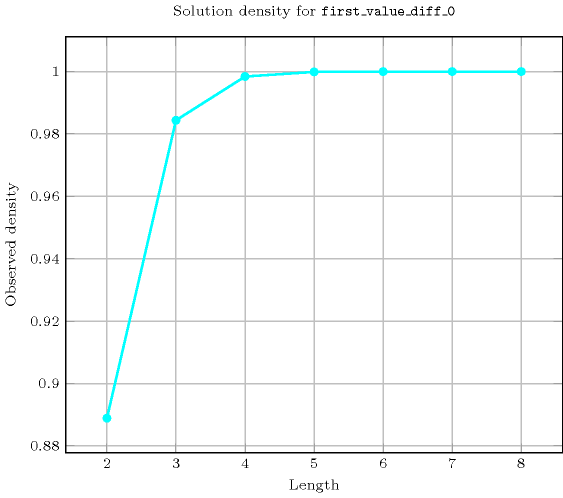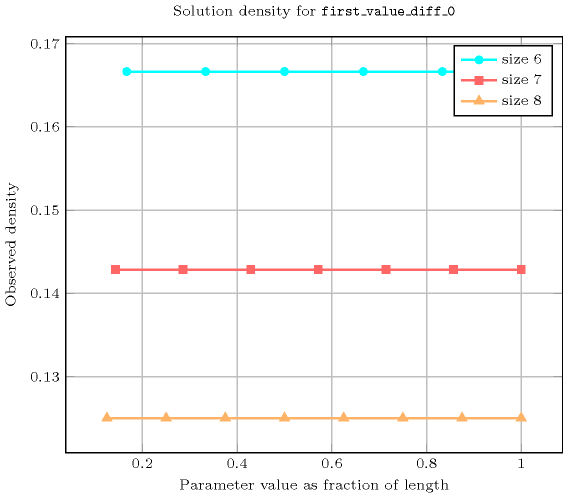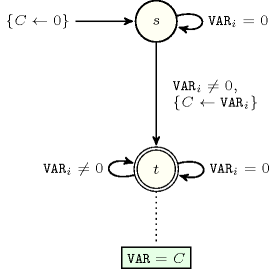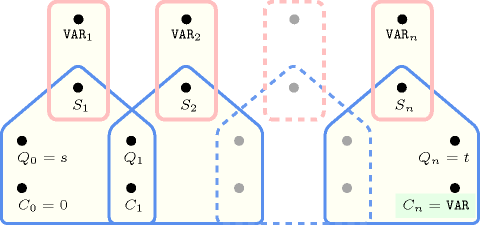## 5.156. first_value_diff_0

Origin

Paparazzi puzzle

Constraint

$\mathrm{𝚏𝚒𝚛𝚜𝚝}_\mathrm{𝚟𝚊𝚕𝚞𝚎}_\mathrm{𝚍𝚒𝚏𝚏}_\mathtt{0}\left(\mathrm{𝚅𝙰𝚁},\mathrm{𝚅𝙰𝚁𝙸𝙰𝙱𝙻𝙴𝚂}\right)$

Synonyms

$\mathrm{𝚏𝚒𝚛𝚜𝚝}_\mathrm{𝚟𝚊𝚕𝚞𝚎}_\mathrm{𝚍𝚒𝚏𝚏}_\mathrm{𝚏𝚛𝚘𝚖}_\mathtt{0}$, $\mathrm{𝚏𝚒𝚛𝚜𝚝}_\mathrm{𝚟𝚊𝚕𝚞𝚎}_\mathrm{𝚍𝚒𝚏𝚏𝚎𝚛𝚎𝚗𝚝}_\mathrm{𝚏𝚛𝚘𝚖}_\mathtt{0}$.

Arguments
 $\mathrm{𝚅𝙰𝚁}$ $\mathrm{𝚍𝚟𝚊𝚛}$ $\mathrm{𝚅𝙰𝚁𝙸𝙰𝙱𝙻𝙴𝚂}$ $\mathrm{𝚌𝚘𝚕𝚕𝚎𝚌𝚝𝚒𝚘𝚗}\left(\mathrm{𝚟𝚊𝚛}-\mathrm{𝚍𝚟𝚊𝚛}\right)$
Restrictions
 $\mathrm{𝚅𝙰𝚁}\ne 0$ $|\mathrm{𝚅𝙰𝚁𝙸𝙰𝙱𝙻𝙴𝚂}|\ge 1$ $\mathrm{𝚛𝚎𝚚𝚞𝚒𝚛𝚎𝚍}$$\left(\mathrm{𝚅𝙰𝚁𝙸𝙰𝙱𝙻𝙴𝚂},\mathrm{𝚟𝚊𝚛}\right)$
Purpose

$\mathrm{𝚅𝙰𝚁}$ is equal to the first non-zero variable of the collection $\mathrm{𝚅𝙰𝚁𝙸𝙰𝙱𝙻𝙴𝚂}$.

Example
 $\left(8,〈0,0,8,0,5〉\right)$ $\left(4,〈4,0,8,0,5〉\right)$
Typical
 $|\mathrm{𝚅𝙰𝚁𝙸𝙰𝙱𝙻𝙴𝚂}|>1$ $\mathrm{𝚖𝚒𝚗𝚟𝚊𝚕}$$\left(\mathrm{𝚅𝙰𝚁𝙸𝙰𝙱𝙻𝙴𝚂}.\mathrm{𝚟𝚊𝚛}\right)<0\vee$$\mathrm{𝚖𝚊𝚡𝚟𝚊𝚕}$$\left(\mathrm{𝚅𝙰𝚁𝙸𝙰𝙱𝙻𝙴𝚂}.\mathrm{𝚟𝚊𝚛}\right)>1$ $|\mathrm{𝚅𝙰𝚁𝙸𝙰𝙱𝙻𝙴𝚂}|-$$\mathrm{𝚊𝚖𝚘𝚗𝚐}_\mathrm{𝚍𝚒𝚏𝚏}_\mathtt{0}$$\left(\mathrm{𝚅𝙰𝚁𝙸𝙰𝙱𝙻𝙴𝚂}.\mathrm{𝚟𝚊𝚛}\right)\ge 1$ $\bigvee \left(\begin{array}{c}|\mathrm{𝚅𝙰𝚁𝙸𝙰𝙱𝙻𝙴𝚂}|\le 4,\hfill \\ |\mathrm{𝚅𝙰𝚁𝙸𝙰𝙱𝙻𝙴𝚂}|-\mathrm{𝚊𝚖𝚘𝚗𝚐}_\mathrm{𝚍𝚒𝚏𝚏}_\mathtt{0}\left(\mathrm{𝚅𝙰𝚁𝙸𝙰𝙱𝙻𝙴𝚂}.\mathrm{𝚟𝚊𝚛}\right)>1\hfill \end{array}\right)$
Arg. properties

Functional dependency: $\mathrm{𝚅𝙰𝚁}$ determined by $\mathrm{𝚅𝙰𝚁𝙸𝙰𝙱𝙻𝙴𝚂}$.

Counting
 Length ($n$) 2 3 4 5 6 7 8 Solutions 8 63 624 7775 117648 2097151 43046720

Number of solutions for $\mathrm{𝚏𝚒𝚛𝚜𝚝}_\mathrm{𝚟𝚊𝚕𝚞𝚎}_\mathrm{𝚍𝚒𝚏𝚏}_\mathtt{0}$: domains $0..n$Length ($n$)2345678
Total8636247775117648209715143046720
 Parameter value

14211561555196082995935380840
24211561555196082995935380840
3-211561555196082995935380840
4--1561555196082995935380840
5---1555196082995935380840
6----196082995935380840
7-----2995935380840
8------5380840

Solution count for $\mathrm{𝚏𝚒𝚛𝚜𝚝}_\mathrm{𝚟𝚊𝚕𝚞𝚎}_\mathrm{𝚍𝚒𝚏𝚏}_\mathtt{0}$: domains $0..n$Figure 5.156.1 depicts an automaton that only accepts all the solutions to the $\mathrm{𝚏𝚒𝚛𝚜𝚝}_\mathrm{𝚟𝚊𝚕𝚞𝚎}_\mathrm{𝚍𝚒𝚏𝚏}_\mathtt{0}$ constraint. This automaton uses a counter in order to record the value of the first non-zero variable ${\mathrm{𝚅𝙰𝚁}}_{i}$ already encountered. To each variable ${\mathrm{𝚅𝙰𝚁}}_{i}$ of the collection $\mathrm{𝚅𝙰𝚁𝙸𝙰𝙱𝙻𝙴𝚂}$ corresponds a 0-1 signature variable ${S}_{i}$. The following signature constraint links ${\mathrm{𝚅𝙰𝚁}}_{i}$ and ${S}_{i}$: ${\mathrm{𝚅𝙰𝚁}}_{i}\ne 0⇔{S}_{i}$.
##### Figure 5.156.1. Automaton (with one counter) of the $\mathrm{𝚏𝚒𝚛𝚜𝚝}_\mathrm{𝚟𝚊𝚕𝚞𝚎}_\mathrm{𝚍𝚒𝚏𝚏}_\mathtt{0}$ constraint##### Figure 5.156.2. Hypergraph of the reformulation corresponding to the automaton (with one counter) of the $\mathrm{𝚏𝚒𝚛𝚜𝚝}_\mathrm{𝚟𝚊𝚕𝚞𝚎}_\mathrm{𝚍𝚒𝚏𝚏}_\mathtt{0}$ constraint